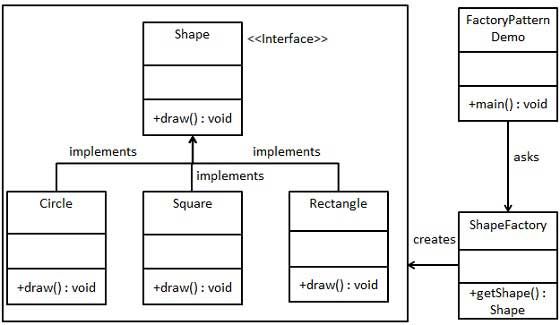﻿

# 工厂模式

## 实现

FactoryPatternDemo，我们的演示类使用 ShapeFactory 来获取 Shape 对象。它将向 ShapeFactory 传递信息（CIRCLE / RECTANGLE / SQUARE），以便获取它所需对象的类型。### 步骤 1

Shape.java

```public interface Shape {
void draw();
}```

### 步骤 2

Rectangle.java

```public class Rectangle implements Shape {

@Override
public void draw() {
System.out.println("Inside Rectangle::draw() method.");
}
}```

Square.java

```public class Square implements Shape {

@Override
public void draw() {
System.out.println("Inside Square::draw() method.");
}
}```

Circle.java

```public class Circle implements Shape {

@Override
public void draw() {
System.out.println("Inside Circle::draw() method.");
}
}```

### 步骤 3

ShapeFactory.java

```public class ShapeFactory {

//使用 getShape 方法获取形状类型的对象
public Shape getShape(String shapeType){
if(shapeType == null){
return null;
}
if(shapeType.equalsIgnoreCase("CIRCLE")){
return new Circle();
} else if(shapeType.equalsIgnoreCase("RECTANGLE")){
return new Rectangle();
} else if(shapeType.equalsIgnoreCase("SQUARE")){
return new Square();
}
return null;
}
}```

### 步骤 4

FactoryPatternDemo.java

```public class FactoryPatternDemo {

public static void main(String[] args) {
ShapeFactory shapeFactory = new ShapeFactory();

//获取 Circle 的对象，并调用它的 draw 方法
Shape shape1 = shapeFactory.getShape("CIRCLE");

//调用 Circle 的 draw 方法
shape1.draw();

//获取 Rectangle 的对象，并调用它的 draw 方法
Shape shape2 = shapeFactory.getShape("RECTANGLE");

//调用 Rectangle 的 draw 方法
shape2.draw();

//获取 Square 的对象，并调用它的 draw 方法
Shape shape3 = shapeFactory.getShape("SQUARE");

//调用 Square 的 draw 方法
shape3.draw();
}
}```

### 步骤 5

```Inside Circle::draw() method.
Inside Rectangle::draw() method.
Inside Square::draw() method.```

﻿